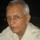maria flor
Branch Unspecified
02 May 2012

# math probs

Can somebody help me in finding the solution of this question???
what is the coefficient of the term next to 792x^9 y^3
a. 2376 b.2640 c.1782 d.2718

thanks!!6 years ago
Frankly speaking, I didn't get the question. Help me recall basic math! 😔maria flor

Branch Unspecified
6 years ago
(ax+by)^12---the equation
[12!(ax)^9 (bx)^3] / 9!3! = 792x^9 y^3
[12!(ax)^8 (bx)^4] / 8!4! = ????6 years ago
maria flor
Can somebody help me in finding the solution of this question???
what is the coefficient of the term next to 792x^9 y^3
a. 2376 b.2640 c.1782 d.2718 thanks!!
wolframalpha can help with all calculations.
https://www.wolframalpha.com/input/?i=(ax +by)^12 =